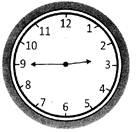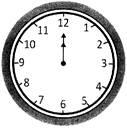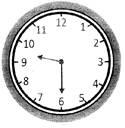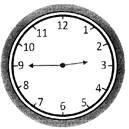Courses

# Test: Time And Calender - 1

## 10 Questions MCQ Test Mathematics (Maths) for Class 3 | Test: Time And Calender - 1

Description
This mock test of Test: Time And Calender - 1 for Class 3 helps you for every Class 3 entrance exam. This contains 10 Multiple Choice Questions for Class 3 Test: Time And Calender - 1 (mcq) to study with solutions a complete question bank. The solved questions answers in this Test: Time And Calender - 1 quiz give you a good mix of easy questions and tough questions. Class 3 students definitely take this Test: Time And Calender - 1 exercise for a better result in the exam. You can find other Test: Time And Calender - 1 extra questions, long questions & short questions for Class 3 on EduRev as well by searching above.
QUESTION: 1

Solution:

40 minutes

QUESTION: 2

Solution:
QUESTION: 3

### Which one among the following statements is  true?

Solution:
QUESTION: 4

Time shown by a clock is 1: 15. It is also read as __________

Solution:
QUESTION: 5

Directions: In each of the questions given below, a clock is shown. You have to identify the time shown in the clock.Solution:
QUESTION: 6

Directions: In each of the questions given below, a clock is shown. You have to identify the time shown in the clock.Solution:
QUESTION: 7

In a clock, hour hand takes how many rounds of the clock face to complete a day?

Solution:
QUESTION: 8

Number of rounds in a day taken by minute hand of a clock is

Solution:
QUESTION: 9

Directions: In each of the questions given below, see the clock and identify the incorrect time given below:Solution:
QUESTION: 10

Directions: In each of the questions given below, see the clock and identify the incorrect time given below:Solution: